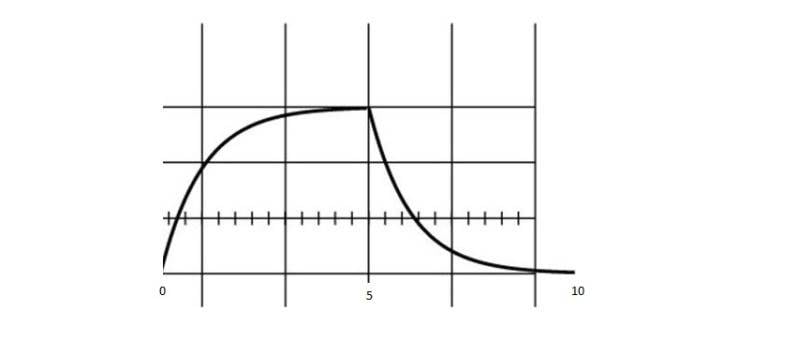# Rms voltage for capacitor charge/discharge

dimpledur

## Homework Statement

Hello,
I was wondering if anyone could confirm my work for the following graph:I'm supposed to find the rms for 1 complete cycle (0, 10ms)

The time constant is 1ms.
Charging phase, v=1-e^(-t/1ms)=1-e^(-1000t)
discharging phase, v=e^(-1000t)

## The Attempt at a Solution

First, I find the rms contribution for the charging stage.
$$\sqrt{\frac{1}{10^{-3}}\int ^{5(10)^{-3}}_{0}{(1-e^{-1000t})^2dt}}=\sqrt{\frac{1}{10^{-3}}(t-\frac{e^{-2000t}}{2000}+\frac{e^{-1000t}}{500}|^{5(10)^{-3}}_{0})}=0.59274 V$$
Next, I found the contribution of the discharge phase:
$$\sqrt{\frac{1}{10^{-3}}\int ^{5(10)^{-3}}_{0}{(e^{-1000t})^2dt}}=\sqrt{\frac{1}{10^{-3}}(-\frac{e^{-2000t}}{2000}|^{5(10)^{-3}}_{0})}=0.707091 V$$
and the effective rms for the entire cycle is
$$v_{rms}=\sqrt{(0.59274 V)^2+(0.707091 V)^2}=0.923 V$$

Or do I have to multiply each of the functions underneatht he the sqrt by a factor of 5(10)^-3 ?

Last edited:

Mentor
Add the means (over the cycle length) of the voltage-squared contributions of each section of the curve prior to taking the square root. So:
$$A = \frac{1}{10^{-3}}\int ^{5(10)^{-3}}_{0}{(1-e^{-1000t})^2dt}$$
$$B = \frac{1}{10^{-3}}\int ^{5(10)^{-3}}_{0}{(e^{-1000t})^2dt}$$
$$v_{rms} = \sqrt{A + B}$$

dimpledur
Interesting. That seems a lot easier. Thanks!

dimpledur
When I do as you stated, I get an rms value of 2V. Steady state voltage in this particular instant is 1V.

Mentor
Oops. The period of the waveform is 10ms or 10-2s, not 10-3s. So the constant multiplying the integrals should be 1/10-2 = 100.

I think you'll find that the RMS value should be 0.634V.

dimpledur
I probably should have caught that. Thanks!